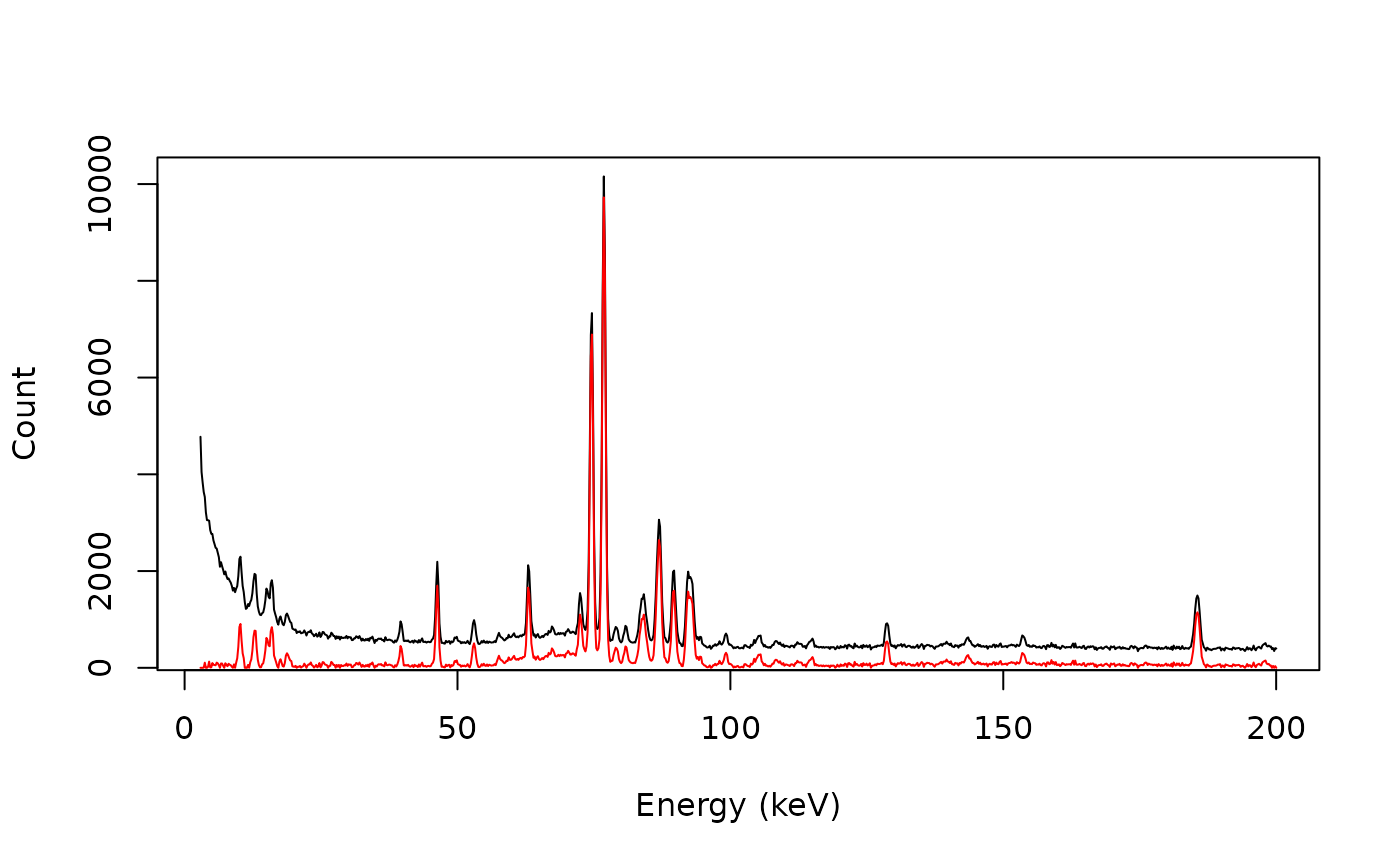Baseline Correction

## Usage

signal_correct(x, y, ...)

# S4 method for numeric,numeric
signal_correct(x, y, method = c("linear", "rubberband", "SNIP", "4S"), ...)

# S4 method for ANY,missing
signal_correct(x, method = c("linear", "rubberband", "SNIP", "4S"), ...)

## Arguments

x, y

A numeric vector. If y is missing, an attempt is made to interpret x in a suitable way (see grDevices::xy.coords()).

...

Extra arguments to be passed to baseline_*() (see details).

method

A character string specifying the method for baseline estimation. It must be one of "linear", "rubberband", "SNIP" or "4S" (see details). Any unambiguous substring can be given.

## Value

Returns a list with two components x and y.

## Details

Available methods for baseline estimation:

linear

Linear baseline estimation (see baseline_linear()).

rubberband

Rubberband baseline estimation (see baseline_rubberband()).

SNIP

Sensitive Nonlinear Iterative Peak clipping algorithm (see baseline_snip()).

4S

4S Peak Filling (see baseline_peakfilling()).

Other signal processing methods: signal_bind(), signal_drift(), signal_mean(), signal_shift(), subset()

N. Frerebeau

## Examples

## gamma-ray spectrometry
data("BEGe")

## Subset from 2.75 to 200 keV
BEGe <- signal_select(BEGe, from = 3, to = 200)

## Drift
baseline <- baseline_snip(BEGe)
BEGe_drif <- signal_drift(BEGe, lag = baseline, subtract = TRUE)

plot(BEGe, type = "l", xlab = "Energy (keV)", ylab = "Count")
lines(BEGe_drif, type = "l", col = "red")## Correct
BEGe_corr <- signal_correct(BEGe, method = "SNIP")

plot(BEGe, type = "l", xlab = "Energy (keV)", ylab = "Count")
lines(BEGe_corr, type = "l", col = "red")